# Multi-value elements in rules

In this post, we will learn:

## Introduction

Have you ever accidentally overwritten the values of all the parameters when you wrote `PARAM | VAL` as path in Rewrite rule, for example? Or that you accidentally overwrote all values of all image URLs?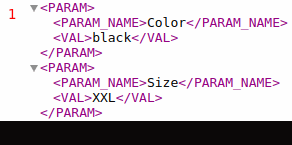What both cases have in common is that they happened by mistake and that they rewrote all the values of some element. In this post, we’ll discuss how this happened and how to properly work with multi-value elements within rules.

## How do rules work with multi-value elements?

### Reading from elements and variables

In some rules, you can work with elements and variables using the % sign: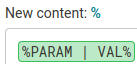If the percent includes a path to a simple element or a variable that has a simple element selected as an input, i.e. only one value is read from, there is nothing to deal with and only the value is worked with. For example, the ITEM_ID element is usually unique within a product: `%ITEM_ID%`.

But what if there is a multi-value element listed among the percentages (so it has more than one value within a single product)? How does the rule know what value to work with? For example, it’s common for parameters to have multiple of them within a single product. We can use the example from introduction as an example: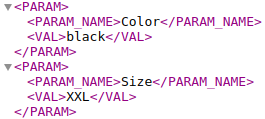The product has 2 parameters. And if we type `%PARAM | VAL%`, then 2 values, namely “black” and “XXL”, match the specified path, since the PARAM element is multi-value.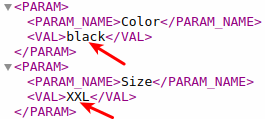So we no longer have just one value, but two. And which one do we want to work with - the first or the second? The rule doesn’t find that out on its own, so the default behavior of the rules is set so that if the rules read from multiple values, they take the first value and continue to work only with it. If we know specifically which value we want to work with, we have to tell the rule - to specify the path to the element, use conditions.

For example, if we only want to take the value of the Size parameter, we would type the following path: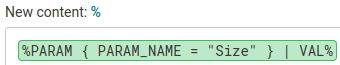This way the rule will only read from parameters that have the value “Size” in the PARAM_NAME element. But note the cases where the product has multiple identical parameters, e.g. Color.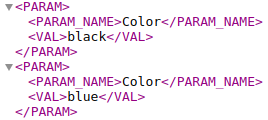Again, it reads from all the parameters that match the path, so for the path `PARAM { PARAM_NAME = "Color" } | VAL`, it reads both “black” and “blue” and takes the first value: “black”.

### Writing to elements

In the previous section, we clarified how rules read from elements - they only take their first value. But what about writing to elements?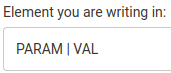Again, as long as the path to the simple element is given in the field, everything is clear. We write the value (which the rule has read, taken, and is working with) into the single value of the element.

But if the field specifies a path to a multi-value element, which value of that multi-value element should the rule write to? The rule itself doesn’t know. And that’s why the default rule behaviour is set so that if rules write to a multi-value element, they write to all of its values.

If we want to write to a specific value, we have to tell the rule that - as we already know, conditions are used to specify the path to the element. For example, if we only want to write to the value of the Color parameter (example from introduction), we just need to specify the path to PARAM_NAME with the value Color: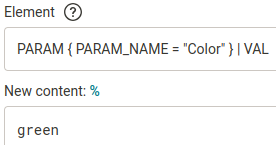This way the rule writes only to parameters that have the value “Color” in the PARAM_NAME element (but beware - it writes the value “green” to all such parameters).MERGADO 1 did not work with multi-value elements, so rules did not work with multi-value elements either, and the described behavior of rules regarding reading and writing values was therefore irrelevant in MERGADO 1.

When writing to elements, one more general rule behavior can be used: When writing to elements - everything that is stated in the condition and is with the operator = or the connecting operator AND, will also be created.

So for example: If we want to add a flag action (currently non-existent) to the Shoptet format, we use the Rewrite rule, in which we enter path in the field for the element:

`FLAGS | FLAG { CODE = "action" } | ACTIVE`

This will add the flag action and the value 0 to it at the same time.

Example: We can add another variant in this way, but the path will be much longer, since the variants have many nested elements:

`VARIANTS | VARIANT { CODE = "ZI1/41" AND EAN = "6655849785123" AND NEGATIVE_AMOUNT = "0" AND AVAILABILITY_IN_STOCK = "In stock" AND UNIT = "pc" AND PARAMETERS | PARAMETER { NAME = "Size" } | VALUE = "EU 41" AND DECIMAL_COUNT = "0" AND AVAILABILITY_OUT_OF_STOCK = "sold out" AND FREE_BILLING = "0" AND APPLY_DISCOUNT_COUPON = "1" AND STOCK | WAREHOUSES | WAREHOUSE { NAME = "Brno" } | VALUE = "0" AND STOCK | WAREHOUSES | WAREHOUSE { NAME = "Prague" } | VALUE = "0" AND FREE_SHIPPING = "0" AND APPLY_QUANTITY_DISCOUNT = "1" AND STANDARD_PRICE = "4500" AND @id = "7575" AND APPLY_VOLUME_DISCOUNT = "1" AND ZBOZI_HIDDEN = "0" AND APPLY_LOYALTY_DISCOUNT = "1" AND PRICE_VAT = "2360" AND HEUREKA_CART_HIDDEN = "0" AND VAT = "21"AND HEUREKA_HIDDEN = "0" AND CURRENCY = "CZK" AND MIN_PRICE_RATIO = "0" AND FIRMY_CZ = "1" AND PRICE_RATIO = "1" AND IMAGE_REF = "imgurl" } | VISIBLE`

### List of rules that read or write

We provide a list of rules that are affected by the reading and writing behavior described above:

RewriteFind and ReplaceBulk Rewrite by QueryBulk Rewrite by ValueBulk Copy Value(Source element)Set product parametersSet shipping ratesSet UTM ParametersCalculationData file import### Warning

In the event that a situation does occur during rule application where the specified path leads to multiple values, the MERGADO Editor will warn the user with a warning. Warnings can tell us that we have made a mistake somewhere or that we have, for example, an unnecessarily large selection in a rule. Read a more detailed post dedicated solely to warnings.By making better selections, we can achieve faster re-generation.

## Example

Let’s take one example: except we’re going to get it right once and wrong the second time.

### Example: correct

Let’s have the following data: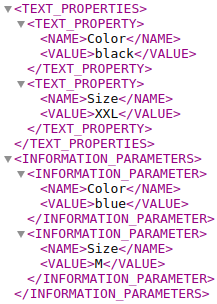Let’s say we would like to have the same color in the information parameter as in the TEXT_PROPERTY parameter. So we create an Overwrite rule that will read from `TEXT_PROPERTIES | TEXT_PROPERTY { NAME = "Color" } | VALUE` and write to `INFORMATION_PARAMETERS | INFORMATION_PARAMETER { NAME = "Color" } | VALUE`: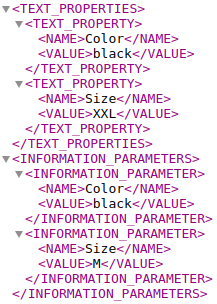So the rule read one value “black” and wrote it to one value “blue”.

### Example: wrong

Let’s have the same data, the same task (to get the value “black” into the value “blue”), and another task: to get the value “XXL” into the value “M”. So actually we want to get all the values of the TEXT_PROPERTY parameter into the information parameter. So we create an Overwrite rule as follows:

After the re-generation we get: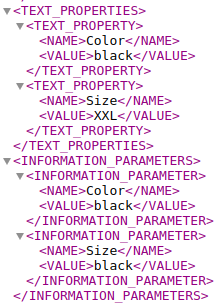And a warning

telling us that multiple values were found for the path `TEXT_PROPERTIES | TEXT_PROPERTY | VALUE`. And indeed, the path references a total of 2 values: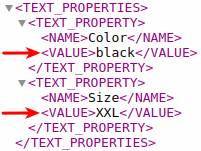So we could specify the path - as in example correct, i.e. `TEXT_PROPERTIES | TEXT_PROPERTY { NAME = "Color" } | VALUE`. At the same time, we should also specify the path to the element where the rule writes (again, see the example correctly), since it currently writes to all values of that element.

Since we also want to get the value of “XXL” into the value of “M”, we’ll create another Overwrite rule and populate it similarly to the first rule, only using Size:

After regenerating the two Rewrite rules created in this way, the values will be correctly assigned to the corresponding values.You can also use the Bulk copy values rule to accomplish both tasks.

## Summary

• How do rules generally work with multi-value elements?
• Rules read from the first value found and write to all values of the multi-value element matching the path. If we want to refine the specified path, we use conditions.
• For single elements, the same applies: the rules read from the first (single) value of the element and write to all (again, single) values of the element. Path refinement is meaningless for simple elements.